﻿
Reach Us+44-7482-875032
Effect of Temperature on Atomic Scattering Curve

# Journal of Applied & Computational MathematicsOpen Access

All submissions of the EM system will be redirected to Online Manuscript Submission System. Authors are requested to submit articles directly to Online Manuscript Submission System of respective journal.

# Effect of Temperature on Atomic Scattering Curve

Haque MM1*, Hasan MM1 and Islam MM2
1Department of Mathematics, Northern University, Dhaka, Bangladesh
2Department of Chemistry, Northern University, Dhaka, Bangladesh
*Corresponding Author: Haque MM, Department of Mathematics, Northern University Bangladesh, Dhaka, Bangladesh, Tel: +8801749951559, Email: [email protected]

Received Date: Jul 29, 2018 / Accepted Date: Sep 12, 2018 / Published Date: Sep 19, 2018

Keywords: Scattering curve; Scattering factor; Temperature factor; Debye temperature; Debye model, MATLLAB software package

#### Introduction

The atomic scattering factor ‘f’ is defined as the ratio of amplitudes: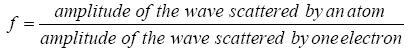It is clear that f=Z (atomic number) for any atom scattering in the forward direction . As θ increases, the waves scattered by individual electrons become more and more out of phase and ‘f’ decreases . The atomic scattering factor depends also on the wavelengths of the incident beam . At a fixed value of θ, ‘f’ will be smaller, the shorter the wavelength, leading to greater interference between the scattered beams. Calculated values of ‘f’ for various atoms and various values of (sin θ)/λ are tabulated in the Tables 1-3. We see that the curve begins at the atomic number and decreases to very low values for scattering in the backward direction (θ near 90º) for very short wavelengths [4-8]. Again we observe that due to temperature factor, the general atomic scattering curve is affected for Debye temperature approximation. From this point of view, we have studied the temperature factor dependence of the atomic scattering curve for Debye temperature approximation [9-11].

 (sinθ)/λ 0 0.1 0.2 0.3 0.4 0.5 0.6 0.7 0.8 0.9 1 1.1 Si (f0) 14 11.35 9.4 8.2 7.15 6.1 5.1 4.2 3.4 2.95 2.6 2.3

Table 1: General Atomic Scattering Factors for Si.

 exp(-M) 1 0.9979 0.9901 0.9744 0.9484 0.9103 0.8592 0.7952 0.7195 0.6345 0.5438 0.4516 f=f0×e-M 14 11.33 9.31 7.99 6.78 5.55 4.38 3.34 2.45 1.87 1.41 1.03

Table 2: when the Debye Approximation temperature factor is considered as (e-M)

 exp(-2M) 1 0.9958 0.9804 0.9494 0.8994 0.8287 0.7383 0.6324 0.5176 0.4025 0.2957 0.2039 f=f0×e-2M 14 11.3 9.22 7.79 6.43 5.06 3.77 2.66 1.76 1.19 0.77 0.47

Table 3: Debye approximation temperature factor is considered as (e-2M)

Thermal (temperature factor) effects on the atomic scattering curve: (Debye approximation)

So far, a crystal has been considered as a collection of atoms located at fixed points in the lattice . Actually, the atoms undergo thermal vibration about their mean positions even at the absolute zero of temperature, and the amplitude of this vibration increases as the temperature increases . We need any quantitative information about the temperature factor e-M, but it is convenient to describe the calculation here before considering other thermal effects. Formally, this effect is included by defining f as the atomic scattering factor of an atom undergoing thermal vibration, f0 as the same quantity for an atom at rest, and the two is related by .

f=f0×e-M (1)

The quantity f0 is then the scattering factor as usually tabulated. Because the intensity of any line depends on f2 calculated intensities must be multiplied by e-M to allow for thermal vibration . The quantity M depends on both the amplitude u of thermal vibration and the scattering angle 2θ: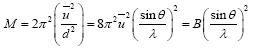(2)

Where, u-2 is the mean square displacement of the atom in a direction normal to the diffracting planes. The exact calculation of u-2 as a function of temperature is extremely difficult , which means that M or B is tough to determine accurately. Peter Debye has given the following expression: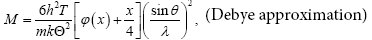(3)

Where, h is the Planck’s constant, T is the absolute temperature, m is the mass of the vibrating atom, k is the Boltzmann’s constant, Θ is the Debye characteristic temperature of the substance in K, x=/T, and ɸ(x) is a function tabulated , along with values of Θ, m=A/N where A=atomic weight and N=Avogadro’s number, the coefficient of the bracketed terms becomes, by inserting the numerical values of the constants in eqn. (3):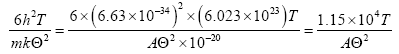If λ is in angstroms. In eqn. (3) is approximate and applies only to elements with cubic crystal structure.

So, ultimately in eqn. (3) reduces to,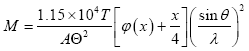(4)

Again, ɸ(x) can be expressed as: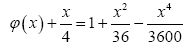Thus, M can be written as,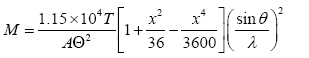(5)

Finally, we get,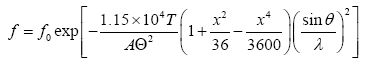(6)

Calculation of general scattering factor

As seen by incident X-ray photons, a sample consists of a spatially inhomogeneous sea of electrons. In fact, quantum mechanics indicates that each electron is itself spread into a diffuse cloud of negative charge. The important quantity is the charge density ρwhich is normally expressed in electron units so that the quantity ρDv is the ratio of charge in volume dv to the charge of one electron. Thus, for each electron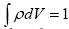. The scattering from a single atom must take into account the phase differences for scattering from different “portions” of the atom, andf0, the atomic scattering factor is made up of the contributions from individual electrons fe given by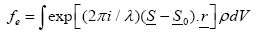Calculation of the precise values of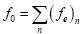depends on the detailed knowledge of the charge distribution ρ.

While it may not be precisely true, assuming that the charge distribution of electrons in an atom is spherical and equals ρ=ρ(r) is a very useful approximation and always a simple expression to be obtained for f0. Using k=(4π/λ) sin θ and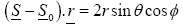and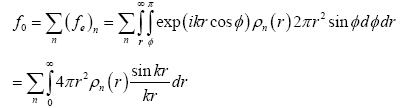(7)

We know that, at small angles, i.e., small k or (sin θ)/λ, approaches unity. If Z is the number of electrons in an atom,

Then,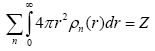(8)

and f0 approaches Z at all small values of sinθ/λ. Thus, the changes in electron density affect the amplitude of scattered x-radiation regardless of the ordering or lack of ordering of the scattering atoms.

A. Calculation of atomic scattering factors for SI

Using the proper charge distribution ρ=ρ(r) of electrons in the atom of Si in eqn.(8), we readily obtain the atomic scattering factors for Si corresponding to(sin θ)/λ, below Table 1.

B. Calculation for scattered atomic scattering factor (Debye approximation)

Here, the equation: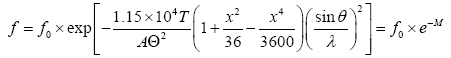For Si, Atomic weight A=28.086, Debye characteristic Temperature Ѳ=645 K, Absolute Temperature T in Kelvin (100, 150, 200, 250, 300, 350, 400, 450, 500, 550, 600, 650).

Using the information obtained in the Tables 1-3, we plot the atomic scattering curve, giving (sinθ)/λ in the x-direction and atomic scattering factors f0 in the y-direction, we obtain the curve:

C. Calculation of atomic scattering factors for Cu

Similarly, using the proper charge distribution ρ=ρ(r) of electrons in the atom of Cu in eqn. (8), we readily obtain the atomic scattering factors for Si corresponding to sinθ/λ, below:

D. Calculation for scattered atomic scattering factor (Debye approximation)

Here, the equation: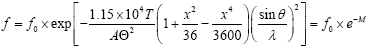For Cu, Atomic weight A=63.546, Debye characteristic Temperature Ѳ=343.5 K, Absolute Temperature T in K (100, 150, 200, 250, 300, 350, 400, 450, 500, 550, 600, 650, 700).

Using the information obtained in the Tables 4-6, we plot the atomic scattering curve, giving (sinθ/λ) in the x-direction and atomic scattering factors f0 in the y-direction, we obtain the curve.

 Sinθ/λ 0 0.1 0.2 0.3 0.4 0.5 0.6 0.7 0.8 0.9 1 1.1 1.2 Cu (f0) 29 25.9 21.6 17.9 15.2 13.3 11.7 10.2 9.1 8.1 7.3 6.6 6

Table 4:  Atomic scattering for Cu.

 exp(-M) 1 0.9974 0.9868 0.9644 0.9266 0.8713 0.7982 0.7092 0.6082 0.5012 0.3951 0.2965 0.2109 f=f0×e-M 29 25.83 21.31 17.26 14.08 11.58 9.34 7.23 5.53 4.05 2.88 1.96 1.27

Table 5: Debye approximation temperature factor is e-M.

 exp(-2M) 1 0.9948 0.9739 0.93 0.8585 0.7592 0.6372 0.5029 0.3699 0.2512 0.1561 0.0879 0.0445 f=f0×e-2M 29 25.77 21.04 16.65 13.05 10.1 7.46 5.13 3.37 2.03 1.14 0.58 0.27

Table 6: Debye approximation temperature factor is e-2M.

E. Calculation of atomic scattering factors for Pt

As above, from eqn. (8), we readily obtain the atomic scattering factors for Pt corresponding to (sinθ)/λ, below:

F. Calculation for scattered atomic scattering factor (Debye approximation)

Here, the equation: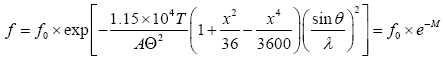For Pt, Atomic weight A=195.08, Debye characteristic Temperature Ѳ=240 K, Absolute Temperature T in Kelvin (100, 150, 200, 250, 300, 350, 400, 450, 500, 550, 600, 650, 700).

Using the information obtained in the Tables 7-9, we plot the atomic scattering curve for Pt, giving (sinθ)/λ in the x-direction and atomic scattering factors f0 in the y-direction, we obtain the curve:

 Sinθ/λ 0 0.1 0.2 0.3 0.4 0.5 0.6 0.7 0.8 0.9 1 1.1 1.2 Pt (f0) 78 72.6 64 56.2 48.9 43.1 39.2 35.6 32.5 29.5 27 24.7 22.7

Table 7:  Atomic scattering for Pt.

 exp(-M) 1 0.9984 0.9915 0.9767 0.9512 0.9133 0.8617 0.7966 0.7192 0.6323 0.5397 0.4458 0.3552 f=f0× exp(-M) 78 72.48 63.46 54.89 46.51 39.36 33.79 28.36 23.37 18.65 14.57 11.01 8.06

Table 8: Debye approximation temperature factor is considered as (e-M)

 exp(-2M) 1 0.9967 0.9831 0.9539 0.9049 0.8341 0.7425 0.6345 0.5173 0.3998 0.2912 0.1987 0.1262 f=f0×e-2M 78 72.36 62.92 53.61 44.25 35.95 29.11 22.59 16.81 11.79 7.86 4.91 2.86

Table 9: Debye approximation temperature factor is considered as (e-2M ).

G. Calculation of atomic scattering factors for C

Similarly, in eqn. (8), we readily obtain the atomic scattering factors for C corresponding to (sinθ)/λ, below:

H. Calculation for scattered atomic scattering factor (Debye approximation)

Here, the equation: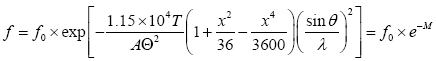For C, Atomic weight A=12.011, Debye characteristic Temperature Ѳ=2230 K, Absolute Temperature T in Kelvin (100, 150, 200, 250, 300, 350, 400, 450, 500, 550, 600, 650).

Using the information obtained in the Tables 10-12 we plot the atomic scattering curve for C, giving (sinθ)/λ in the x-direction and atomic scattering factors f0 in the y-direction, we obtain the curve.

 Sinθ/λ 0 0.1 0.2 0.3 0.4 0.5 0.6 0.7 0.8 0.9 1 1.1 C (f0) 6 4.6 3 2.2 1.9 1.7 1.6 1.4 1.3 1.16 1 0.9

Table 10: Atomic scattering for C.

 exp(-M) 1 1.0019 0.9998 0.9937 0.9845 0.9723 0.9567 0.9377 0.915 0.8882 0.8575 0.8227 f=f0× exp(-M) 6 4.61 3 2.19 1.87 1.65 1.53 1.31 1.19 1.03 0.86 0.74

Table 11: Debye Approximation temperature factor is considered as (e-M).

 exp(-2M) 1 1.0015 0.9995 0.9875 0.9693 0.9453 0.9154 0.8793 0.8371 0.789 0.7353 0.6769 f=f0×e-2M 6 4.6 2.99 2.17 1.84 1.61 1.46 1.23 1.09 0.92 0.74 0.61

Table 12: Debye approximation temperature factor is considered as (e-2M).

#### Results and Discussions

Atomic scattering curve decreases with temperature factor. We have calculated the effect of temperature of the atomic scattering curve of the elements Si, Cu, Pt and C. for the calculation, firstly we have considered the general scattering and then considered the temperature factors (e-M) and (e-2M) and obtain the Figures 1-4, using Debye approximation where Debye temperature factor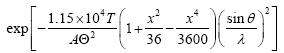and observe that the atomic scattering curve decreases a little bit compared with general scattering curve. So, atomic scattering curve is affected by the temperature factor.

Figure 4: Temperature dependence of atomic scattering curve for C with Debye approximation.

#### References

Citation: Haque MM, Hasan MM, Islam MM (2018) Effect of Temperature on Atomic Scattering Curve. J Appl Computat Math 7: 417. DOI: 10.4172/2168-9679.1000417

Copyright: © 2018 Haque MM, et al. This is an open-access article distributed under the terms of the Creative Commons Attribution License, which permits unrestricted use, distribution, and reproduction in any medium, provided the original author and source are credited.

Select your language of interest to view the total content in your interested language

##### Recommended Journals
Viewmore
###### Article Usage
• Total views: 589
• [From(publication date): 0-0 - Aug 24, 2019]
• Breakdown by view type
• HTML page views: 540
• PDF downloads: 49

## Post your commentCan't read the image? click here to refresh
###### Peer Reviewed Journals

Make the best use of Scientific Research and information from our 700 + peer reviewed, Open Access Journals

International Conferences 2019-20

Meet Inspiring Speakers and Experts at our 3000+ Global Annual Meetings

Top